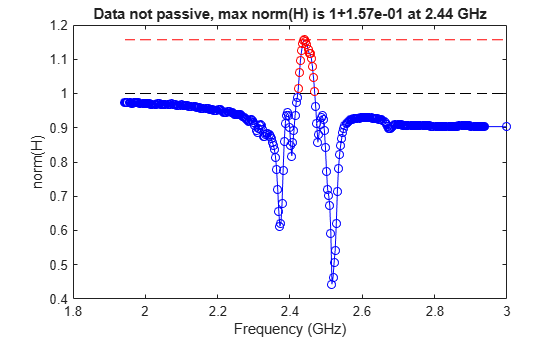# passivity

Plot passivity of S-parameters object

## Syntax

``passivity(s)``
``[ns,violationindex] = passivity(s)``

## Description

example

````passivity(s)` plots the passivity of S-parameter object, `s` over a range of frequencies specified in `s.Frequencies`. Passivity is measured by the two-norm of the frequency-dependent transfer function H(f).```
````[ns,violationindex] = passivity(s)` plots the passivity and returns the data of the passivity plot.```

## Examples

collapse all

Read the file, `sawfilter.s2p`.

`S = sparameters('sawfilter.s2p');`

Plot the passivity of the 2-by-2 S-parameters.

`passivity(S)`## Input Arguments

collapse all

S-parameters, specified as an RF Toolbox™ s-parameter function object. To create this type of object, use the `sparameters` function.

## Output Arguments

collapse all

Two-norm transfer function H(f) at each frequency in `s.Frequencies`, returned as a column vector.

Data Types: `double`

Frequencies indices where passivity is violated (norm(H)>1), returned as a column vector.

Data Types: `double`

## Version History

Introduced in R2019a